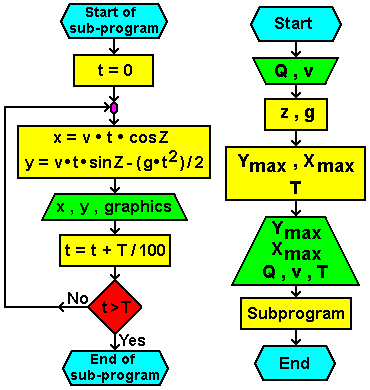# 6.1.6. Range of oblique shot1. phase
Calculate range of oblique shots and its graphical representation.

2. phase
Calculate the range of oblique shots, maximum height and time of flight for the value of the selected object Q angle and initial speed v assuming that the horizon is straight.
The initial speed of the object must not be greater than 1500 m/s.
Based on the calculated data plotted graphically display the weather patterns of the calculation of 1/100 of the total duration of the flight facility.

3. phase
Mathematical equations and associated constants are:

g = 9.80665
PI = 3.141593
---------------------------------------------------
0 < Q < 90°
0 < v < 1500 m/s
Z = (Q•PI) / 180

Ymax = (v2•sin2Z) / (2•g)

T = (Z•v•sinZ) / g

Xmax = (v2•sin(2•Z)) / g

4. phase
Flowchart:Citing of this page: Radic, Drago. " IT - Informatics Alphabet " Split-Croatia. {Date of access}. . Copyright © by Drago Radic. All rights reserved. | Disclaimer